# Iron collecting

Class 7A collected 3.2 tonnes of iron more than class 7B. Together they collected 6.4 tonnes of iron to the secondary raw material collection. How much did each class collect?

Correct result:

a =  4.8 t
b =  1.6 t

#### Solution:

a=3.2 + b
a+b = 6.4

a-b = 3.2
a+b = 6.4

a = 24/5 = 4.8
b = 8/5 = 1.6

Our linear equations calculator calculates it.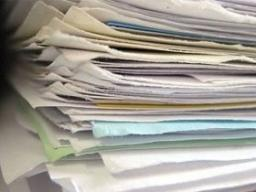We would be pleased if you find an error in the word problem, spelling mistakes, or inaccuracies and send it to us. Thank you!Tips to related online calculators
Do you have a linear equation or system of equations and looking for its solution? Or do you have quadratic equation?

## Next similar math problems:

• The water barrel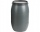The water barrel weighs 122 kg. If we pour 75% of the water out of it, it will weigh 35 kg. What is the weight of the barrel?
• Two teas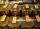A mixture weighing 10 kg was made from two types of tea. The price of tea was 160 CZK/kg and second tea 170 CZK/kg. The price of the mixture is 166 CZK/kg. How many kilograms of each type of tea had to be mixed?
• CoinsThe money - coins are minted from the hardest bronze, which contains copper and tin in a ratio of 41: 9. How much copper and tin are in 2kg of bronze money?
• A bottleA bottle full of cola weighs 1,320 g. If we drink three-tenths of it, it will weigh 1,008g. How much does an empty bottle weigh?
• The cube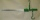The cube and cylinder weigh the same as three 20-gram weights. Three cubes and one cylinder weigh the same as two cylinders and one cube. How many grams does one cube weigh (and one cylinder)?
• Bent scaleMonica weighed 52 kg. Sara 54 kg. Together they weighed 111 kg. They noticed that the weight on the scale was bent. How much did they really weigh?
• Vegetable meal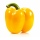The cook was doing meal - in ratio 4: 3: 1 mix tomatoes: pepper: onion. Onions were 5 kg less than peppers. How many kgs of tomatoes did he need to prepare the meal?
• The farm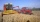The farm harvested 390 tons of grain. Wheat was 15% more than barley, and rye was 126 tons less than wheat and barley combined. How many tons of wheat, how many tons of barley, and how much rye did the team get?The fruit basket is five times heavier than the empty basket. Filled is 20kg heavier than empty. Determine the weight of the fruit in the basket.
• Camel and water84% of the camel's weight is water. After drinking, its weight increased to 832 kg and water accounted for 85% of its weight. How much did it weigh before drinking?
• The devils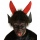The devils weighed in hell with Dorota. They found that Dorota and the two devils weigh 250 kg together and Dorota and the four devils weigh 426 kg. All the devils weigh the same. How Much Does Dorota Weigh?
• Trio weight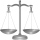Adelka, Barunka, and Cecilka are weight in pairs. Adelka with Barunka weighs 98 kg, Barunka with Cecilka 90 kg and Adelka with Cecilka 92 kg. How much does each of them weigh?
• PotatoesFor three days the store sold 1400 kg of potatoes. The first day they sold 100 kilograms of potatoes less than the second day, the third-day three-fifths of what they sold the first day. How many kgs of potatoes sold every day?
• Luggage and air travelTwo friends traveling by plane had a total of 35 kg of luggage. They paid one 72 CZK and second 108 CZK for being overweight. If only one paid for all the bags, it would cost 300 CZK. What weight of baggage did each of them have, how many kilograms of lug
• CranberriesThey brought 65 kg of dried cranberries to the packing house. They made 150-gram and 200-gram packages from them. How many kilograms of cranberries did they use for the smaller 200 packages? How many 200 gram packages were there?
• BucketThe bucket half filled with water weighs 5.55 kg, the full bucket weighs 9.85 kg. How much does the bucket weigh?
• Father and sonFather and son weigh together 108kg. The father weighs 2.6 times more than his son. How much does weight my father and son count?